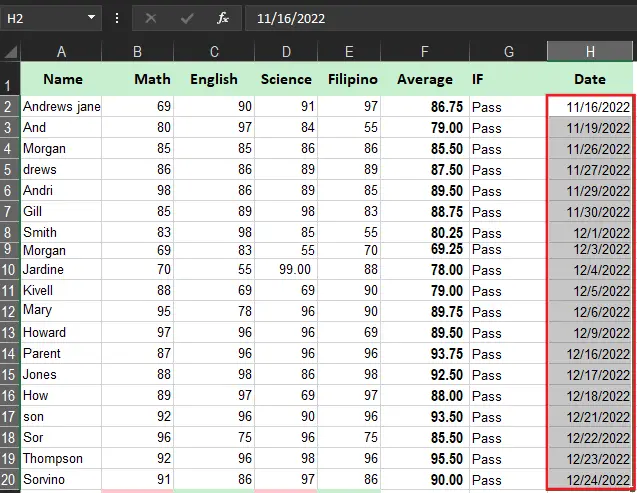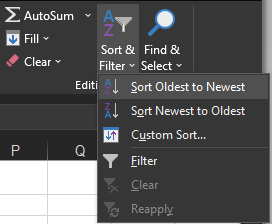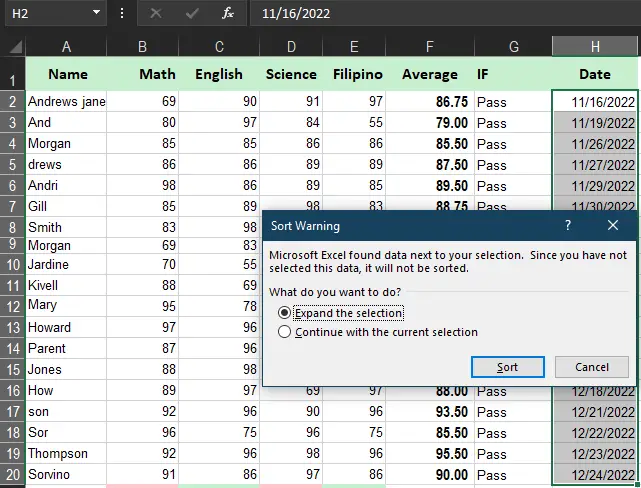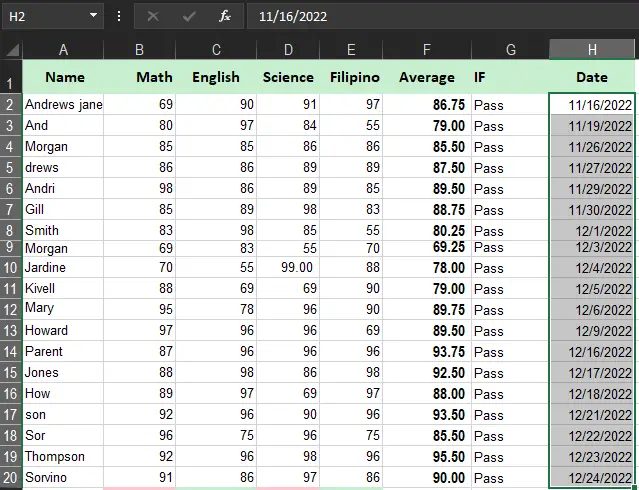How To Sort By Date In Excel with Examples and Formula

# How To Sort By Date In Excel with Examples and Formula

In this tutorial, we will discover how to sort by date in Excel, along with different criteria such as weekday, month and year. Moreover with such examples includes sorting of different format of dates.

Stay tuned as we unlock this new learning.

## What is Sorting Excel by date?

Sorting dates in Excel is one of the most beneficial features which allows us to sort dates based on our preferred format. Basically, there are different entries of data we could have, such as data wherein ascending or descending order of year, month or week, or day.

Apparently, it could be useful in planning or arranging schedules, getaways, or even transactions revenue, or sorting weekly budgets.

To get through all of that let’s proceed on how it may apply in every instance thus how to actually work on it.

## How to sort by date chronological order in Excel

Time needed: 3 minutes.

Let’s say we already have data that consists of dates and ready to work on. Further, Microsoft Excel provided tools to make it simpler.
So here are the things we all need to do:

1. Select the column of list of dates you want to sort chronologically.2. Go to HOME Tab and Editing group.3. Select the Sort and Filter then sort Oldest to Newest

The dialog box will display with an option Expand the selection or Continue with the current selection4. Expand the selection or Continue with the current selection

However, if there are data in the adjacent column select Expand the selection otherwise select continue with the current selection. In our case will expand the selection.5. Another option is you can select the A-Z on the Data Tab in the Sort and filter group.

## Sort by Weekday date Excel

There are also instances where we sort data by weekday. To do this we need a helper column, so here will manipulate the helper column along with WEEKDAY formula. Hence it returns the corresponding day of the week and then sorts by the helper column.

If the list of data starts with Sunday(1) ends with Saturday(7) the formula use is this:

`=WEEKDAY(H2)`

However, if the data starts on Monday(1) and end on Sunday(7) the formula to be used is this:

`=WEEKDAY(H2, 2)`

Hence the H2 is the cell that contains the date in the image below we are using the first formula mentioned above.

## Excel date sort by month

We more likely know that it is inevitable to know what month the data is entered, as a result we will sort by month in Excel. One instance is when collecting all the dates when the students submitted their requirements. So we can determine who meets the deadline.

Moreover in this scenario month is significant while the year is irrelevant.

We know that Excel has a lot of functions and formulas we can use and one of these is the MONTH function. This function is used to pull data entries into full dates.

Do the following:

2. Click the empty cell in the month column.n this case, it will be I2. Type =MONTH(H2), where H2 refers to the first entry in the Date column.

Generally, the corresponding cell will return the number of months from 1 to 12. So these numbers represent the chronological order of months. Namely, 1 is January and 12 is December.

To enter the function quickly into every cell, select the cell that contains the formula. In this case, it’s I2. Then, click and drag the green box that appears in the bottom corner of the cell, all the way to the bottom of the column.

## Excel sorting by date month and day

Excel provides sorting of date by month and day, this is one of the solutions in certain instances where you want to arrange dates and birthdays. Therefore this feature will take months and days.

Moreover, the Excel Text function is alternatively used to perform this function wherein it converts a string to a preferred format thus making it easier. For our purpose, the “mmdd” or “mm.dd” format code will work.

With the source date in B2, the formula takes this form:

So the date in cell H2 will use this formula:

`=TEXT(H2, "mm.dd")`

Next, sort the Month and day column from largest to smallest, and you will have the data arranged in order of the days of each month.

Afterwards, just sort the data from smallest to largest then you will have the data which sort on the days of each month.

Additionally, it will obtain when using the DATE formula, take on a look below:

`=DATE(2022, MONTH(H2),DAY(H2))`

So this formula yields a list of dates from month and day based on their actual which is placed on Cell H2 wherein replaces the real year with a fake one.

The idea is to have the same year for all the dates, and then sort the list of dates in chronological order. Since the year is the same, the dates will be sorted by month and day, which is exactly what you are looking for.

## Excel date sorting year

You’re almost done guys! looks how smart you are. Now you know what would be next.

Well, we will discuss this function carefully and briefly. You are right the year function is like the first sorting we learned. when we sort from largest to smallest or oldest to newest the Mircosoft Excel sorts dates with year too.

Therefore if you are observant in manipulating formulas, you already know how to use the year function:

`=YEAR(B2)`

So when your columns of date is ready, you do the same process. Sort smallest to largest and choose expand selection in the dialog box.

So here you can see data sorted by year.

## Excel custom sorting month names entered as text

If there are instances that your date is specified as text and not formatted as date, therefore displaying this in ascending order might be a trouble.

Hence, Excel can custom sort month names entered as text just follow the following:

1. Choose the data of month name you want to sort.
2. Click Sort on the Data tab in the Sort and Filter group.
3. When the sort dialog box appears do the following:
4. In your Column list dialog box select the full months names (JanuaryFebruaryMarch, …)or ther abbreviation name (JanFebMar…) it still depends on you how you want to appear in your worksheet.
5. To close the dialog box Click OK twice.

## Excel sort by date not working

Apparently, if all the formulas and examples above do not return the sorting of dates correctly, you evaluate and input again the format you have entered so that Microsoft Excel will recognize it. Possibly it is stored as text therefore it can not be recognized.

In addition to fixing this, kindly click the text dropdown menu located in the Excel Ribbon and choose the date format.

## Summary

As a recap on the article How To Sort By Date In Excel with Examples and Formula, we learned that there are a lot of ways to in sorting dates, formulas and techniques.

I hope this tutorial in Excel helps you a lot. See you in our next tutorial!